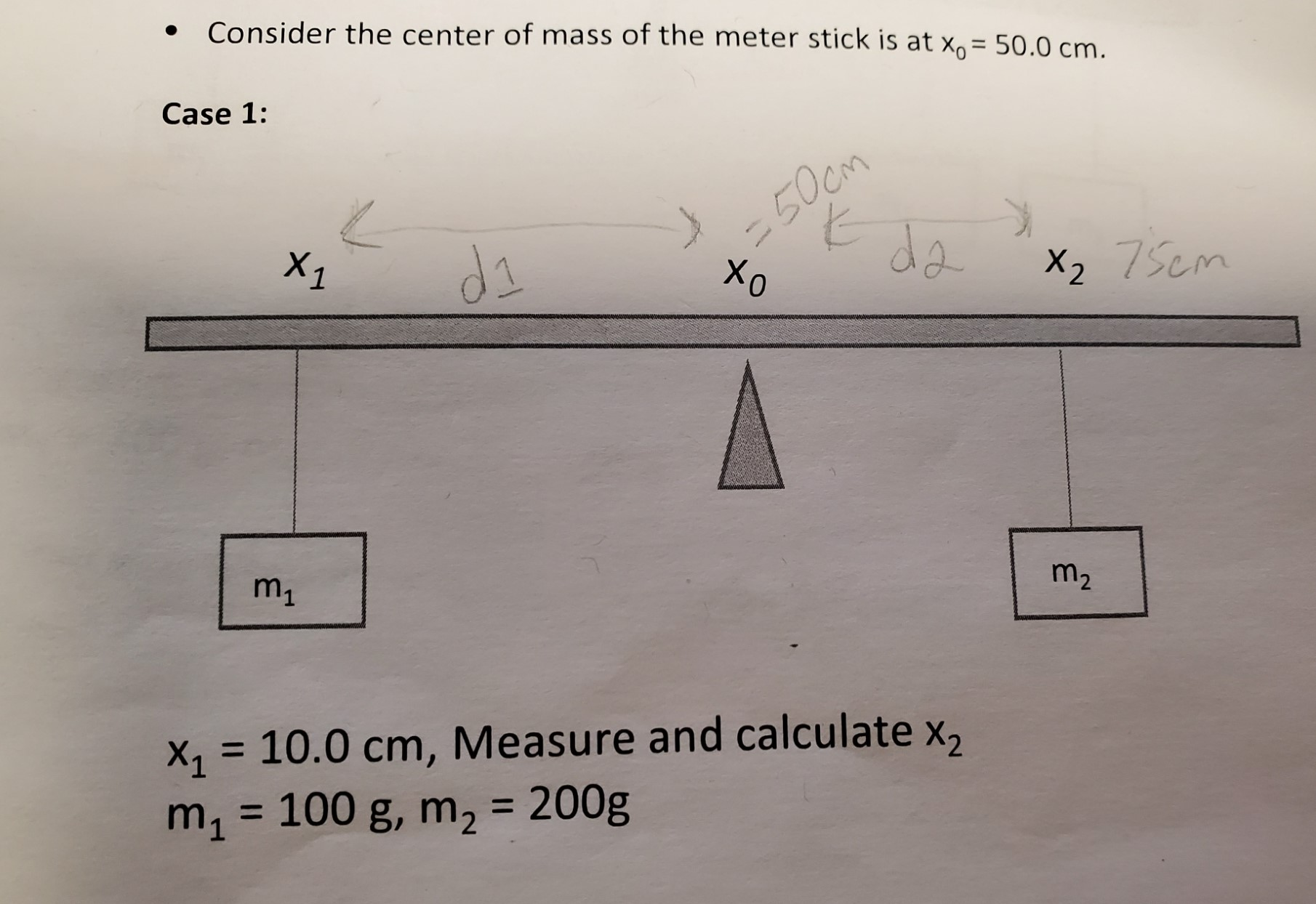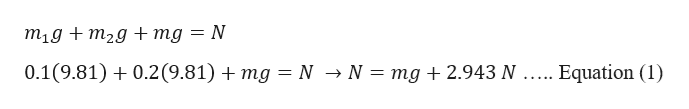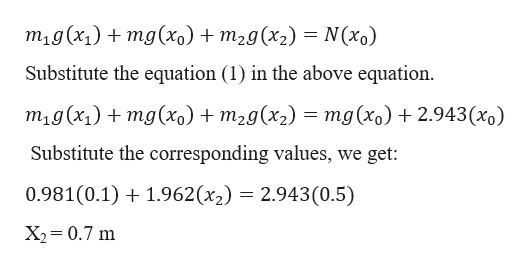# Consider the center of mass of the meter stick is at x= 50.0 cm.Case 1:-50cmdaX1diX2 75cmm1m210.0 cm, Measure and calculate x,100 g, m2 = 200gmX1

Question

Explain how the condition (sum)F = 0 is satisfied for the meter stick in case 1 of the experiment.

Case 1: In Picture.help_outlineImage TranscriptioncloseConsider the center of mass of the meter stick is at x= 50.0 cm. Case 1: -50cm da X1 di X2 75cm m1 m2 10.0 cm, Measure and calculate x, 100 g, m2 = 200g m X1 fullscreen
check_circle

star
star
star
star
star
1 Rating
Step 1

Given information:

Mass of the block (m1) = 100 g = 0.1 kg

Mass of the block (m2) = 200 g = 0.2 kg

Let the mass of the stick be “m”

Measure of x1 is given as = 10 cm = 0.1 m

Measure of x2 is =?

Step 2

For the meter stick to be in equilibrium the sum of the forces in the vertical direction is zero i.e.help_outlineImage Transcriptionclosem1g+m2g + mg = N 0.1(9.81)0.2(9.81) mg = N > N = mg + 2.943 N ..... Equation (1) fullscreen
Step 3

For the meter stick to be in equilibrium the net moment should also ...help_outlineImage Transcriptionclosem1g(x1)mg(X0) + m2g(x2) = N(x0) Substitute the equation (1) in the above equation mig (x1)mg(x) + m2g(x2) = mg(xo) 2.943(x Substitute the corresponding values, we get: 0.981(0.1)1.962(x2) = 2.943(0.5) X2 0.7 m fullscreen

### Want to see the full answer?

See Solution

#### Want to see this answer and more?

Solutions are written by subject experts who are available 24/7. Questions are typically answered within 1 hour.*

See Solution
*Response times may vary by subject and question.
Tagged in

### Newtons Laws of Motion